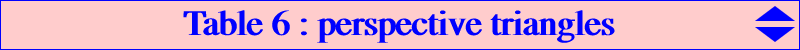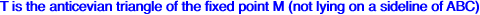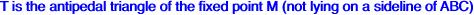Let P be a point in the plane of the reference triangle ABC and denote by cevP : the cevian triangle of P acvP : the anticevian triangle of P pedP : the pedal triangle of P apdP : the antipedal triangle of P ccvP : the circumcevian triangle of P cacP : the circumanticevian triangle of P refP : the reflection triangle of P in the sidelines of ABC symP : the reflection triangle of P in the vertices of ABC. cevP* : the cevian triangle of the isogonal conjugate P* of P, and similarly for the other triangles The following tables give the loci of P such that two of these triangles are perspective. Note the frequent occurence of the Darboux cubic K004. true means the triangles are perspective for any P, true(H) means the triangles are actually homothetic L denotes the line at infinity, C denotes the circumcircle, 6B the union of the six bisectors.ABC cevP acvP pedP apdP ccvP cacP refP cevP true acvP true true pedP K004 union of the altitudes K004 apdP K004 K004 C, 6B K004 ccvP true true true L, C and K003 true cacP union of the symmedians union of the symmedians true a nonic L, C and the sextic Q028 true see Table 34 refP K001 K060 true true(H) L, C and K006 C and K003 a nonic symP true(H) true true L and K243 L, C and K001 true L and the quintic Q029 L and K004cevP acvP pedP apdP ccvP cacP refP symP cevP* 6B true three conics, see Table 11 L, C and K004 C and a septic C and a septic a sextic L and a quintic acvP* true 6B K004 C and 6B C and Q026 K102 and a nK(X6,X2) Q075 L and a quintic pedP* three nodal cubics, see Table 11 K004 three isogonal focal pK true(H) C and 10th degree 12th degree a nonic L and a circular septic apdP* L, C and K004 L, C and 6B true(H) L, C and 6B L, C and K003 L, C and a sextic true(H) L and a circular quintic ccvP* L and an octic L and a quintic L and an octic L, C and K003 C, 6B and K024 L and a quintic L and an octic L and a sextic cacP* a quintic K102 and a nK(X6,X2) a nonic L, C and a sextic C and Q026 6B and a sextic a nonic L and an octic refP* a nonic Q075 a nonic true(H) C and 10th degree 12th degree K006 L and a bicircular septic symP* C and a septic C and a septic C and a quintic L, C and a septic L and a circular sextic C and 10th degree C and Q067 true(H)Loci related to refP perspective with a fixed triangle T This section written with Martin Acosta's cooperation In this section we study the locus L(P) of P such that refP is perspective with a FIXED given triangle T and, if possible, the locus L(Q) of the perspector Q. Several loci are already available in the table above when T is the reference triangle ABC.Let T = A1B1C1. Denote by A2, B2, C2 the reflections of A1 in BC, B1 in CA, C1 in AB respectively. Let A3 = BC2 /\ CB2 and define B3, C3 similarly. The locus L(P) is a circular circum-cubic which contains M, A2, B2, C2, A3, B3, C3. L(P) also contains A1 if and only if M lies on the isogonal pK with pivot the midpoint of the altitude AH. Hence, L(P) contains the three points A1, B1, C1 if and only if M is an in/excenter. When M = I, the cubic is K269 = pK(X6, X515) and when M is an excenter, it is the isogonal pK whose pivot is the corresponding extraversion of X(515), the infinite point of the line IH. L(P) is a K0 (i.e. a cubic without term in xyz) if and only if M lies on the Napoleon cubic K005, and in this case, it is always a pK. This is the case of K269. ***Let T = A1B1C1. Denote by A2, B2, C2 the reflections of A1 in BC, B1 in CA, C1 in AB respectively. Let A3 = BC2 /\ CB2 and define B3, C3 similarly. The locus L(P) is a circular circum-cubic which contains M* = isogonal conjugate of M, A2, B2, C2, A3, B3, C3. L(P) also contains A1 if and only if M lies on the isogonal pK with pivot the antipode of A on the circumcircle. Hence, L(P) contains the three points A1, B1, C1 if and only if M is an in/excenter. When M = I, the cubic is K269 = pK(X6, X515) and when M is an excenter, it is the isogonal pK whose pivot is the corresponding extraversion of X(515), the infinite point of the line IH. L(P) is a K0 (i.e. a cubic without term in xyz) if and only if M lies on K270 = pK(X6, X1503).Loci related to circumperp triangles The circumperp triangles CP1, CP2 and their tangential triangles TCP1, TCP2 are defined in Clark Kimberling's TCCT (§§ 6.21 upto 6.23). The vertices of CP1 (resp. CP2) are the second intersections of the circumcircle with the external (resp. internal) bisectors of ABC. The following table gives the loci of P such that a triangle related to P is perspective with one of these four triangles.CP1 CP2 TCP1 TCP2 cevP line X1-X7 and circumconic with perspector X(1) pK(X81, X86)=K317 pK(X1252, X4998) through X(2), X(55), X(100), X(1252), X(4998) pK(X593, X261)=K320 acvP line X1-X6 and circumconic with perspector X(55) pK(X1333, X81)=K319 pK(X16283, X2) through X(2), X(55), X(1376) pK(X5019, X2)=K321 pedP a non-circum cubic through X(1), X(3), X(1490) a non-circum cubic through X(1), X(3) a non-circum cubic through X(3) a non-circum cubic through X(3) apdP L, C, and pK(X1333, X21)=K318 L, C and the line X1-X3 L, C and a cubic through X(4), X(56), X(945) L, C and a cubic through X(3), X(4), X(55), X(64) ccvP C, external bisectors and line X1-X3 C, internal bisectors and line X36-X238 C, line X1-X3 and a cubic C, line X36-X238 and pK(X1333, X21)=K318 cacP internal bisectors and a cubic external bisectors and pK(X1333, X81)=K319 line X1-X6, circumconic with perspector X(55) and a cubic pK(X1333, X81)=K319 and another cubic refP a circular non-circumcubic through X(1), X(3), X(101), X(515), X(993) a focal non-circumcubic through X(1), X(3), X(36), X(109), X(515) a circular non-circumcubic through X(3), X(30) a circular non-circumcubic through X(3), X(30) symP L and a conic through X(382), X(1482) L and a conic through X(1), X(21), X(382), X(1482) true trueThese triangles CP2 and CP1 can be seen as the circumcevian and circumanticevian triangles of the incenter. This latter point may be replaced by any other fixed point Q as far as it does not lie on a sideline or on the circumcircle. The most interesting generalization is obtained with ccvQ, the circumcevian triangle of Q, since we always find three related pK. The following table gives the loci of P such that ccvQ is perspective to cevP, acvP (or cacP) and also the locus of the perspector which is the same for all these triangles.pole of the pK pivot of the pK isopivot of the pK cevP p^2 / [a^2(c^2q+b^2r)] : : p / [a^2(c^2q+b^2r)] : : Q acvP a^2 / (c^2q+b^2r) : : 1 / (c^2q+b^2r) : : K = X(6) persp. a^2 / (c^2q+b^2r) : : (-a^2qr+b^2rp+c^2pq) / (c^2q+b^2r) : : a^2 / (-a^2qr+b^2rp+c^2pq) : :Loci related to other triangles The following table gives another selection of loci related to several triangles as in Clark Kimberling TCCT p.155 & sq and other "classical" triangles.Cevian and Anticevian, Pedal and Antipedal triangles of a fixed point QCevian triangle Anticevian triangle Pedal triangle Antipedal triangle cevP cevian lines of Q true 3 lines sidelines acvP true cevian lines of Q sidelines 3 lines pedP 3 lines perpendicular to the sidelines of ABC at the vertices of the cevian triangle of Q K004 (see note below) 3 lines L, C and a circum-cubic apdP L, C and K004 L, C and 3 lines L, C and a circum-cubic L, C and cevian lines of Q ccvP C and a circum-quartic through Q sidelines, a line and C C and a circum-quartic C, sidelines and a line cacP trilinear polar of Q and a quintic sidelines, a line and a conic a circum-sextic sidelines, C and a circum-cubic refP a cubic a circum-cubic a cubic L, C and a circular circum-cubic symP L and a conic L and a conic L and a conic C and a conicNote : for any Q and for any P on K004, pedP and acvQ are perspective and the locus of the perspector is a cubic which is the transform of the Lucas cubic K007 under the mapping M -> M/Q (M-Ceva conjugate of Q). This cubic is a pK in acvQ with pivot the isogonal of Q in the orthic triangle and isopivot X(69)/Q. When Q = H, it is also a pK in ABC.Intangents and Extangents triangles details in TCCT §6.16 p.161 and §6.17 p.162Intangents triangle Extangents triangle cevP Feuerbach hyperbola K033 = pK(X37, X8) acvP Circum-hyperbola with perspector X(663) passing through X(6), X(9), X(19), etc K362 = pK(X213, X1) pedP Central cubic with center X(1) passing through X(4), X(40), X(944) Central cubic with center X(40) passing through X(4), X(40) apdP L, C and a circum-cubic through X(3), X(40), X(84) L, C and pK(X3 x X942, X1) passing through X(1), X(3), X(58), X(500), X(501), X(942) ccvP C and a circum-quartic through X(4), X(84), X(365) C and a circum-quartic through X(4), X(55), X(65), X(365) cacP Circum-sextic through X(2), X(57), X(365) Circum-sextic through X(2), X(6), X(365) refP L and a conic through X(1), X(4), X(19), X(221) L and a conic through X(4), X(33), X(40), X(55), X(199) symP L and a rectangular hyperbola through X(40), X(2574), X(2575) L and a rectangular hyperbola through X(1), X(65), X(71), X(2574), X(2575)Reflected triangles A'B'C' is the triangle formed by the reflections of A, B, C in the sidelines of ABC. The hexyl triangle is the triangle formed by the reflections of the excenters in the circumcenter O of ABC. A1B1C1 is the triangle formed by the reflections of O in the vertices of ABC (TCCT §6.13).A'B'C' Hexyl A1B1C1 cevP K060 K344 a pK through X(2), X(3), X(5), X(69), X(1173), X(1994) acvP K005 K343 a pK with pivot G through X(2), X(3), X(6), X(1656) pedP K127 a cubic through X(1), X(1498) a cubic through X(3), X(20), X(382) apdP K364 L, C and K343 L, C and a cubic through X(3), X(4), X(64), X(1657) ccvP C and a circum-quartic through H C and a quartic through X(1), X(3), X84), X(513) C and a circular circum-quartic through X(3), X(1173), X(2574), X(2575) cacP circum-sextic through K and X(1989) a sextic through X(1), X(6), X(57) a sextic through X(6), X(288) refP K060 a cubic through X(1), X(515) a cubic through X(3), X(30), X(382), X(2080) symP L and a rectangular hyperbola through H, K, X(1657), X2574), X(2575) L and a conic through X(1), X(84) trueSee other loci related to the four Brocard triangles. See also K585, K586 for cubics related to the Morley triangle.Loci related to cevian and anticevian triangles The following table gathers together a good number of pKs, the loci of P whose cevian / anticevian triangle is perspective at Q to a certain triangle T. Note that the locus of the perspector Q is the same pK in both cases. This is a direct adaptation of César Lozada's tables in ETC, see X(8782) and X(8856), with some addional informations.pKs related to cevian triangle of P and with anticevian triangle of P Triangle T pK Pole Pivot pK Pole Pivot Locus of Q 1st anti-Brocard K738 76 3978 K739 385 6 K699 anti-McCay -- 598 8785 -- 8859 8860 1st Brocard K322 1916 694 K128 6 385 K020 2nd Brocard K531 3455 67 K534 3 524 K538 3rd Brocard K532 8789 694 K128 6 385 K020 4th Brocard K533 8791 8791 K535 25 468 K539 5th Brocard -- 3051 141 -- 16285 2 pK(16285,2896) circummedial -- 308 308 K644 251 83 K959 circumorthic -- 8794 8795 -- 8882 275 K919 2nd circumperp K317 81 86 K319 1333 81 K318 Euler -- 8796 8797 K671 53 2 pK(53,3091) 2nd Euler -- 2 317 -- 216 6515 K044 5th Euler -- 8801 8801 K517 427 4 extangents K033 37 8 K362 213 1 K750 2nd extouch K007 2 69 K033 37 8 K880 3rd extouch K007 2 69 K964 1427 7 K963 4rd extouch -- 8813 8814 -- 1214 7 pK(1214,5933) 5th extouch -- 8816 8817 -- 8898 7 Feuerbach -- 8818 3615 K672 115 1 K877 outer-Garcia K366 321 75 K345 37 2 K033 inner-Grebe -- 494 5491 -- 6421 2 outer-Grebe -- 493 5490 -- 6422 2 hexyl K344 81 8822 K343 6 63 K004 Johnson K674 324 264 K612 216 2 K044 Kosnita -- 2 311 -- 571 1994 K388 Lucas central -- 8825 588 -- 8908 6 Lucas tangents -- 8950 493 -- 8911 6 McCay -- 8587 8827 -- 6 8859 midheight K007 2 69 K004 6 20 K002 mixtilinear -- 8828 8829 -- 198 57 3rd mixtilinear -- 7366 269 -- 604 57 4th mixtilinear -- 1253 9 -- 31 1 pK(31,165) 5th mixtilinear K365 57 7 -- 9 2 K201 6th mixtilinear K202 1 144 K351 6 9 pK(6,2951) Morley K586 3275 5456 K585 6 3602 K029 inner-Napoleon K420a 14 8836 K129a 6 395 K005 outer-Napoleon K420b 13 8838 K129b 6 396 K005 1st Neuberg -- 3407 8840 K128 6 385 K422 2nd Neuberg -- 1916 8842 K423 6 3329 K422 orthocentroidal K060 1989 265 K001 6 30 K005 reflection K060 1989 265 K005 6 5 K001 1st Sharygin K132 1 894 K673 1914 6 K960 2nd Sharygin K323 1 239 K673 1914 6 K961 inner-squares K070b 4 1585 K006 6 4 K424a outer-squares K070a 4 1586 K006 6 4 K424b inner-Vecten K070a 4 1586 K424b 6 3069 K006 outer-Vecten K070b 4 1585 K424a 6 3068 K006